# Discrete Mathematics Using a Computer

Computer science abounds with applications of discrete mathematics, yet s- dents of computer science often study discrete mathematics in the context of purely mathematical applications. They have to ?gure out for themselves how to apply the ideas of discrete mathematics to computing problems. It is not easy. Most students fail to experience broad success in this enterprise Computer science abounds with applications of discrete mathematics, yet s- dents of computer science often study discrete mathematics in the context of purely mathematical applications. They have to ?gure out for themselves how to apply the ideas of discrete mathematics to computing problems. It is not easy. Most students fail to experience broad success in this enterprise, which is not surprising, since many of the most important advances in science and engineeringhavebeen, precisely, applicationsofmathematicstospeci?cscience and engineering problems. Tobesure, mostdiscretemathtextbooksincorporatesomeaspectsapplying discrete math to computing, but it usually takes the form of asking students to write programs to compute the number of three-ball combinations there are in a set of ten balls or, at best, to implement a graph algorithm. Few texts ask students to use mathematical logic to analyze properties of digital circuits or computer programs or to apply the set theoretic model of functions to understand higher-order operations. A major aim of this text is to integrate, tightly, the study of discrete mathematics with the study of central problems of computer science

Compare

Computer science abounds with applications of discrete mathematics, yet s- dents of computer science often study discrete mathematics in the context of purely mathematical applications. They have to ?gure out for themselves how to apply the ideas of discrete mathematics to computing problems. It is not easy. Most students fail to experience broad success in this enterprise Computer science abounds with applications of discrete mathematics, yet s- dents of computer science often study discrete mathematics in the context of purely mathematical applications. They have to ?gure out for themselves how to apply the ideas of discrete mathematics to computing problems. It is not easy. Most students fail to experience broad success in this enterprise, which is not surprising, since many of the most important advances in science and engineeringhavebeen, precisely, applicationsofmathematicstospeci?cscience and engineering problems. Tobesure, mostdiscretemathtextbooksincorporatesomeaspectsapplying discrete math to computing, but it usually takes the form of asking students to write programs to compute the number of three-ball combinations there are in a set of ten balls or, at best, to implement a graph algorithm. Few texts ask students to use mathematical logic to analyze properties of digital circuits or computer programs or to apply the set theoretic model of functions to understand higher-order operations. A major aim of this text is to integrate, tightly, the study of discrete mathematics with the study of central problems of computer science

## 30 review for Discrete Mathematics Using a Computer

1.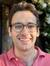4 out of 5

Cesare De Cal

I rented this textbook from my university library without knowing what to expect. It’s exceptionally clear and accurate, a true masterpiece that’s helped me immensely to pass the exam. The use of the Haskell language is the icing on the cake. Thanks for writing such a great book.

2.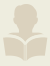4 out of 5

Jonathan

Rex Page helped write this book.

3.4 out of 5

Oliver Dechant

4.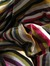5 out of 5

Mattias Lundell

5.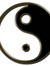4 out of 5

Jose_A.

6.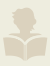5 out of 5

Conscell

7.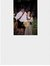4 out of 5

Steve Graham

8.4 out of 5

John

9.4 out of 5

rev

10.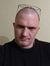5 out of 5

Andy Legkiy

11.4 out of 5

12.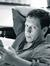5 out of 5

Hugo Sereno Ferreira

13.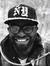5 out of 5

Jason Gordon

14.4 out of 5

Shahbaz

15.5 out of 5

Chris

16.4 out of 5

K

17.4 out of 5

Paul Smith

18.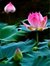4 out of 5

Nguyen Ai

19.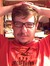4 out of 5

Alexey Vyskubov

20.5 out of 5

K.

21.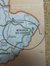5 out of 5

Miranda Edwards

22.4 out of 5

Nikita

23.5 out of 5

Ruhi

24.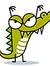4 out of 5

Gabriel Sohappy

25.4 out of 5

Cesar

26.5 out of 5

ashir kuniyil

27.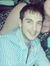4 out of 5

Cristian

28.5 out of 5

Pratik Chaudhari

29.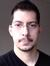4 out of 5

Jrabelo

30.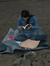5 out of 5

Tae